Next: Nonlinear state-space models Up: Learning algorithms Previous: Learning algorithms   Contents

### Ensemble learning for hidden Markov models

MacKay showed in  how ensemble learning can be applied to learning of HMMs with discrete observations. With suitable priors for all the variables, the problem can be solved analytically and the resulting algorithm turns out to be a rather simple modification of the Baum-Welch algorithm.

MacKay uses Dirichlet distributions as priors for the model parameters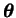. (See Appendix A for the definition of the Dirichlet distribution and some of its properties.) The likelihood of the model is discrete with respect to all the parameters and the Dirichlet distribution is the conjugate prior of such a distribution. Because of this, the posterior distributions of all the parameters are also Dirichlet. The update rule for the parameters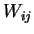of the Dirichlet distribution of the transition probabilities is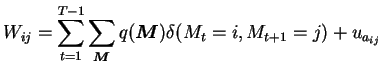(4.11)

where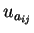are the parameters of the prior and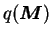is the approximate posterior used in ensemble learning. The update rules for other parameters are similar.

The posterior distribution of the hidden state probabilities turns out to be of exactly the same form as the likelihood in Equation (4.6) but with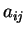replaced with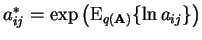and similarly for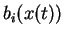and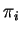. The required expectations over the Dirichlet distribution can be evaluated as in Equation (A.13) in Appendix A.Next: Nonlinear state-space models Up: Learning algorithms Previous: Learning algorithms   Contents
Antti Honkela 2001-05-30